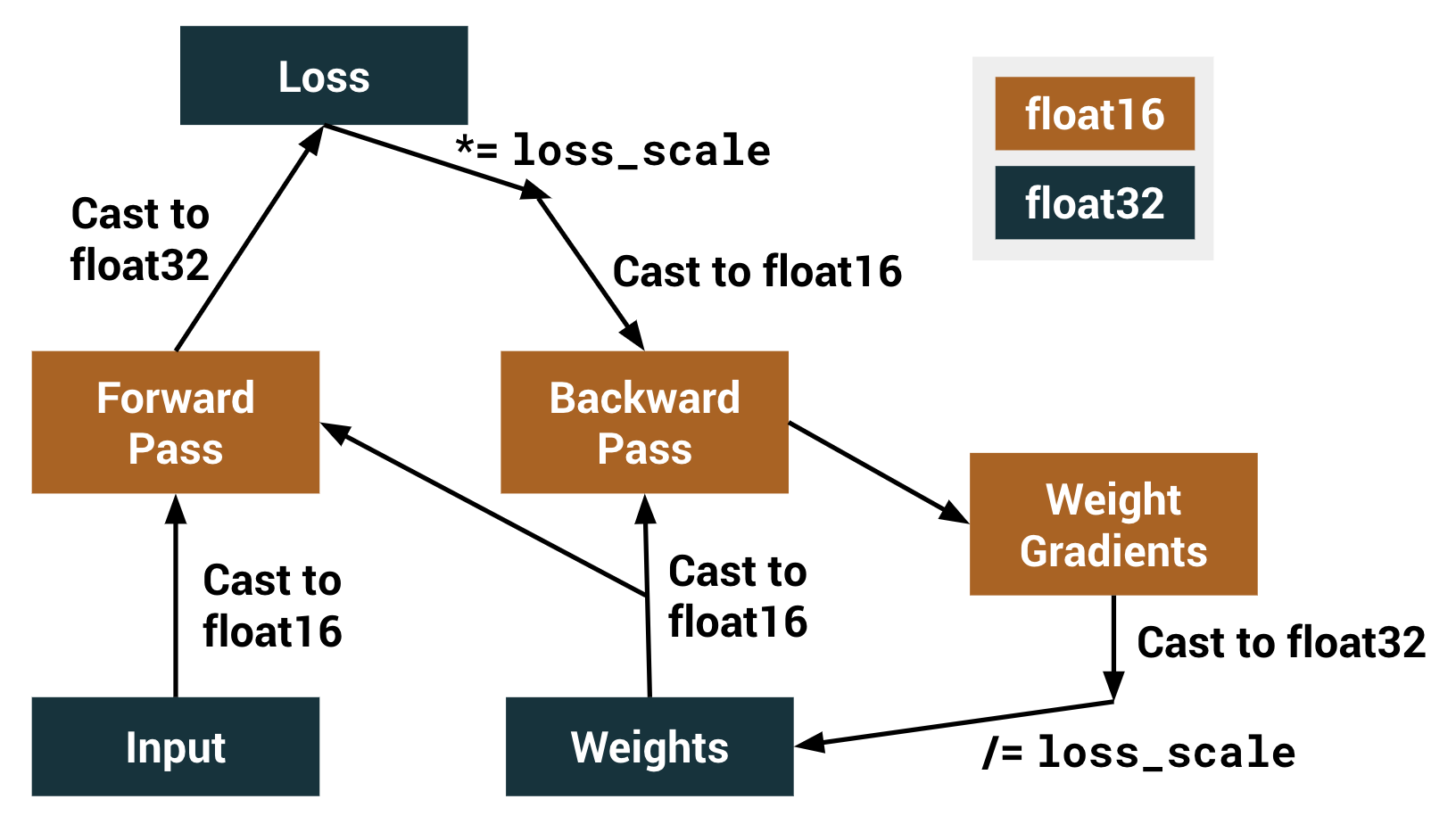Missed TensorFlow Dev Summit? Check out the video playlist.

# tf.compat.v1.train.experimental.enable_mixed_precision_graph_rewrite

Enable mixed precision via a graph rewrite.

``````tf.compat.v1.train.experimental.enable_mixed_precision_graph_rewrite(
opt, loss_scale='dynamic'
)
``````

Mixed precision is the use of both float32 and float16 data types when training a model to improve performance. This is achieved via a graph rewrite operation and a loss-scale optimizer.

Performing arithmetic operations in float16 takes advantage of specialized processing units, such as NVIDIA Tensor Cores for much higher arithmetic throughput. However, due to the smaller representable range, performing the entire training with float16 can result in gradient underflow, that is, small gradient values becoming zeroes. Instead, performing only select arithmetic operations in float16 results in higher throughput and decreased training time when using compatible hardware accelerators while also reducing memory usage, typically without sacrificing model accuracy.

#### Example:

``````model = tf.keras.models.Sequential([
...
])

opt = tf.keras.optimizers.SGD()
opt = tf.train.experimental.enable_mixed_precision_graph_rewrite(opt)

model.compile(loss="categorical_crossentropy",
optimizer=opt,
metrics=["accuracy"])

model.fit(x_train, y_train,
batch_size=batch_size,
epochs=epochs)
``````

For a complete example showing the speed-up on training an image classification task on CIFAR10, check out this Colab notebook.

Calling `enable_mixed_precision_graph_rewrite(opt)` enables the graph rewrite operation before computing gradients. The function additionally returns an `Optimizer`(`opt`) wrapped with a `LossScaleOptimizer`. This prevents underflow in the float16 tensors during the backward pass. An optimizer of type `tf.train.Optimizer` or `tf.keras.optimizers.Optimizer` must be passed to this function, which will then be wrapped to use loss scaling.The graph rewrite operation changes the `dtype` of certain operations in the graph from float32 to float16. There are several categories of operations that are either included or excluded by this rewrite operation. The following categories of Ops are defined inside corresponding functions under the class `AutoMixedPrecisionLists` in auto_mixed_precision_lists.h:

• `ClearList`: Ops that do not have numerically significant adverse effects. E.g. `ArgMax` and `Floor`.
• `WhiteList`: Ops that are considered numerically safe for execution in float16, and thus are always converted. E.g. `Conv2D`.
• `BlackList`: Ops that are numerically unsafe to execute in float16 and can negatively affect downstream nodes. E.g. `Softmax`.
• `GrayList`: Ops that are considered numerically safe for execution in float16 unless downstream from a BlackList Op. E.g. `Add` and `AvgPool`.

When this function is used, gradients should only be computed and applied with the returned optimizer, either by calling `opt.minimize()` or `opt.compute_gradients()` followed by `opt.apply_gradients()`. Gradients should not be computed with `tf.gradients` or `tf.GradientTape`. This is because the returned optimizer will apply loss scaling, and `tf.gradients` or `tf.GradientTape` will not. If you do directly use `tf.gradients` or `tf.GradientTape`, your model may not converge due to float16 underflow problems.

When eager execution is enabled, the mixed precision graph rewrite is only enabled within `tf.function`, as outside `tf.function`, there is no graph.

For NVIDIA GPUs with Tensor cores, as a general performance guide, dimensions (such as batch size, input size, output size, and channel counts) should be powers of two if under 256, or otherwise divisible by 8 if above 256. For more information, check out the NVIDIA Deep Learning Performance Guide.

Currently, mixed precision is only enabled on NVIDIA Tensor Core GPUs with Compute Capability 7.0 and above (Volta, Turing, or newer architectures). The parts of the graph on CPUs and TPUs are untouched by the graph rewrite. TPU support is coming soon. CPUs are not supported, as CPUs do not run float16 operations faster than float32 operations.

#### Raises:

`ValueError` when `mixed_precision_global_state.using_default_mixed_precision_policy` is set to `False` before `tf.train.experimental.enable_mixed_precision_graph_rewrite()` is called.

#### Returns:

A version of `opt` that will use loss scaling to prevent underflow.